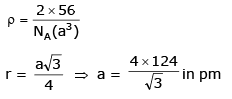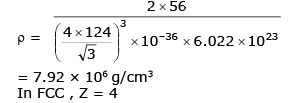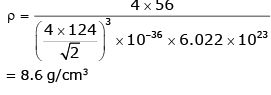Courses

# Solid State MCQ - 1 (Advanced)

## 25 Questions MCQ Test Chemistry for JEE Advanced | Solid State MCQ - 1 (Advanced)

Description
This mock test of Solid State MCQ - 1 (Advanced) for JEE helps you for every JEE entrance exam. This contains 25 Multiple Choice Questions for JEE Solid State MCQ - 1 (Advanced) (mcq) to study with solutions a complete question bank. The solved questions answers in this Solid State MCQ - 1 (Advanced) quiz give you a good mix of easy questions and tough questions. JEE students definitely take this Solid State MCQ - 1 (Advanced) exercise for a better result in the exam. You can find other Solid State MCQ - 1 (Advanced) extra questions, long questions & short questions for JEE on EduRev as well by searching above.
*Multiple options can be correct
QUESTION: 1

### Select the correct statement (s)

Solution:

(A) 6 Cs+ ion second nearest neighbour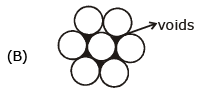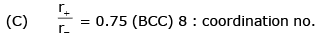(D) True

*Multiple options can be correct
QUESTION: 2

### The compound having general formula M2+ Fe2O42_ are called as :

Solution: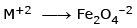it will be ferrite and ferrimagnetic substance

*Multiple options can be correct
QUESTION: 3

### Which of the following statement is/are incorrect in the rock-salt structure if an ionic compounds ?

Solution:

Coordination no. of cation = 6
coordination no of anion = 6

*Multiple options can be correct
QUESTION: 4

Which of the following statements are correct :

Solution:

r+ + r = a/2
(95 + 181)= a/2
⇒ a = 276 × 2
a = 552 pm

*Multiple options can be correct
QUESTION: 5

Passage

In two dimension, there are square close packing & Hexagonal close packing. In three dimension, however threre are HCP, BCC and CCP arrangement. Unoccupied space in packing is known as void which may be octahedral, tetrahedral or cubic void and formation of void depends on atomic or ionic arrangment in crystal.

Q.

Empty space left in HCP in three dimension is

Solution:

H C P
Pacling efficlency = 74%
empty space = 100 – 74%
ES=26%

*Multiple options can be correct
QUESTION: 6

In two dimension, there are square close packing & Hexagonal close packing. In three dimension, however threre are HCP, BCC and CCP arrangement. Unoccupied space in packing is known as void which may be octahedral, tetrahedral or cubic void and formation of void depends on atomic or ionic arrangment in crystal.

Q.

In ccp, ratio of no. of atoms to no. of tetrahedral void is -

Solution:

In any structure if number of atom is Z
so no. of tetrahedral voids = 2 Z
in ccp Z = 4
so letrahedral void = 8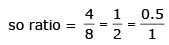*Multiple options can be correct
QUESTION: 7

In two dimension, there are square close packing & Hexagonal close packing. In three dimension, however threre are HCP, BCC and CCP arrangement. Unoccupied space in packing is known as void which may be octahedral, tetrahedral or cubic void and formation of void depends on atomic or ionic arrangment in crystal.

Q.

Size order of octahedral, tetrahedral & cubic void is -

Solution:

For B.C.C.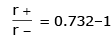for octahedral.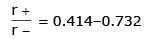for tetrahedral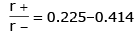so, cubic > octahedral > tetrahedral

*Multiple options can be correct
QUESTION: 8

In two dimension, there are square close packing & Hexagonal close packing. In three dimension, however threre are HCP, BCC and CCP arrangement. Unoccupied space in packing is known as void which may be octahedral, tetrahedral or cubic void and formation of void depends on atomic or ionic arrangment in crystal.

Q.

In certain metallic oxide, metal M occupy HCP lattice & O_2 occupy 2/3rd of octahedral void, formula of crystal is -

Solution:

M— H.C.P = 6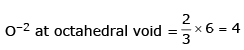M6O4
M3O2

*Multiple options can be correct
QUESTION: 9

Statement - I. Due to Frenkel defect the density of solid decrease.

Statement - II. In Frenkel defect cation or anion is present at interstitial site leaving lattice sites vacant.

Solution:

A Frenkel defect is a type of defect in crystalline solids wherein an atom is displaced from its lattice position to an interstitial site, creating a vacancy at the original site and an interstitial defect at the new location within the same element without any changes in chemical properties.

No cation are anion are leaving the crystal. Only the cation is moving from one place to another place within the crystal. So the density of crystalline solid will not change.

*Multiple options can be correct
QUESTION: 10

Statement - I. By increasing the temperature C.N. of CsCl changes from 8 : 8 to 6 : 6.

Statement - II. Temperature also influences the C.N. of solid.

Solution:

CSCl has b.c.c
by increasing temperature it will become octahedral type and it C.N. will be 6
Staterment - I is correct and statement II is correct explanation of I So answer will be (A)

*Multiple options can be correct
QUESTION: 11

Statement - I. Solid having more F-centers have intense color.

Statement - II. Excess of Na+ in NaCl solid having F-centers make it appears to pink.

Solution:

statment I is correct
because F- certres are the electron occupied at the place of anion which is released by metal thus it will give intense colour whereas statement II is wrong

*Multiple options can be correct
QUESTION: 12

Statement - I. Close packing of atoms in cubic structure is in order of : fcc > bcc > sc

Statement - II. Packing density =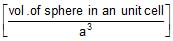Solution:

F.C.C. = 74%
B.C.C. = 68%
SC= 52%
So FCC > BCC > SC
So statement I is correct
Statement II correct.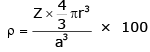and correct explanation of I

*Multiple options can be correct
QUESTION: 13

Statement - I. Pure NaCl is diamagnetic in nature.

Statement - II. In NaCl, Na+ occupy octahedral voids.

Solution:

Statement I - No unpaired electron in NaCl so diamagnetic
Statemetn II - is correct but not correct exblanation of I so

QUESTION: 14

State whether the following statements are True or False :

Coluor of LiCl due to metal excess defect is pink.

Solution:

In case we caused oping lithium goes in interstitiel site whcih cause pink colour True.

QUESTION: 15

State whether the following statements are True or False :

The imperfection in crystal increases with increases in temperature.

Solution:

As we increase the temperature effect will also increase.

QUESTION: 16

State whether the following statements are True or False :

FCC structure possesses the most close packing of atoms in it.

Solution:

FCC and ccp has the highest packing efficiency

QUESTION: 17

State whether the following statements are True or False :

Increase in pressure in ionic solids may decrease co-ordination No.

Solution:

Increase in pressure increase coordination number

QUESTION: 18

State whether the following statements are True or False :

The amorphous solids are isotropic.

Solution:

The amporsphous solid are isotropic.

QUESTION: 19

COLUMN MATCHING :

Match the following columns :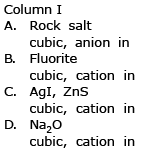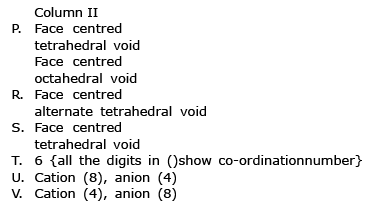Solution:

(A) Rock salt
Na+Cl → F.C.C & cation in octahedral void.
T. C.N –6.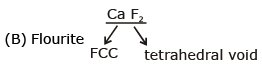So PU.
(C) AgI zns — R W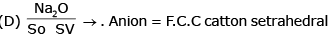vidi C.N. 4 & 8

QUESTION: 20

A cubic solid is made up of two elements A and B. Atoms B are at the corners of the cube and A at the body centre. What is the formula of compound.

Solution:

A = 1 (Body center)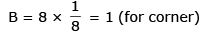formula = AB

QUESTION: 21

A compound alloy of gold and copper crystallizes in a cubic lattice in which gold occupy that lattice point at corners of the cube and copper atom occupy the centres of each of the cube faces. What is the formula of this compound.

Solution: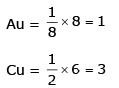Formula = AuCu3

QUESTION: 22

A cubic solid is made by atoms A forming close pack arrangement, B occupying one. Fourth of tetrahedral void and C occupying half of the octahedral voids. What is the formula of compound.

Solution: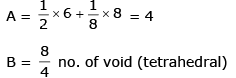= 2
C = Total no. of octahedral voids / 2 no. of octahedral voids (effective) = 1 + 12 × 1/4 = 4
Bodycenter   edgecentre C = 4/2 = 2
formula = A4B2C2

QUESTION: 23

What is the percent by mass of titanium in rutile, a mineral that contain Titanium and oxygen, if structure can be described as a closet packed array of oxide ions, with titanium in one half of the octahedral holes. What is the oxidation number of titanium ?

Solution: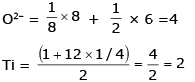TiO2 formula
Ti (At mass) = 47.88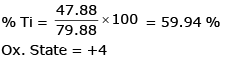QUESTION: 24

Spinel is a important class of oxides consisting of two types of metal ions with the oxide ions arranged in CCP pattern. The normal spinel has one-eight of the tetrahedral holes occupied by one type of metal ion and one half of the octahedral hole occupied by another type of metal ion. Such a spinel is formed by Zn2+, Al3+ and O2-, with Zn2+ in the tetrahedral holes. Give the formulae of spinel.

Edge length, density and number of atoms per unit cell

Solution:

Zn2+ = 8/8 = 1
Al3+ = 4/2 = 2
O2– = CCP (4)
ZnAl2O4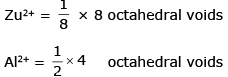O2– = CCP arrangment (1+3) = 4

QUESTION: 25

Iron occurs as bcc as well as fcc unit cell. If the effective radius of an atom of iron is 124 pm. Compute the density of iron in both these structures.

Solution:

In bcc z = 2